The NIST reference dataset contains 27 datasets for validating nonlinear regression analysis software. The datasets were distributed by level of difficulty: 8 lower, 11 average and 8 higher. Here, will be presented the LAB Fit results for the lower level. For each dataset a function is indicated and the initial values are suggested (two groups). The two groups were tested by LAB Fit. For most of the datasets, the uncertainty could be given with 1 or 2 significant digits. However, the LAB Fit results were presented with 3 digits for the uncertainty. The value 1 was assumed for the "POWER" parameter and for the tolerance was assumed the default value: 1.0E-06. Although LAB Fit calculates the covariance matrix (for error propagation purposes, confidence and prediction bands), the results won't be presented because the SRD / NIST doesn't certify it.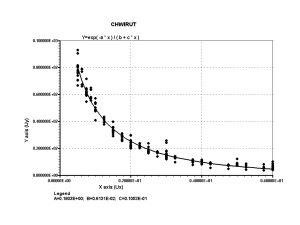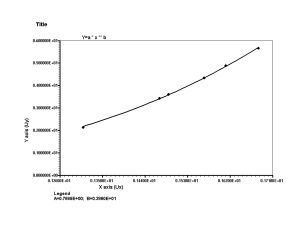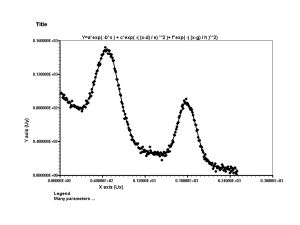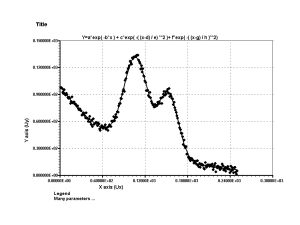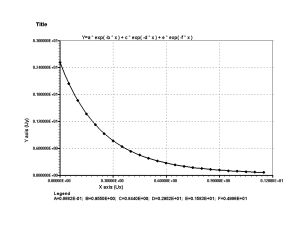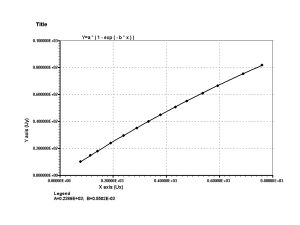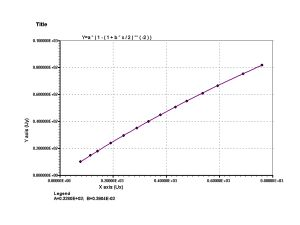CHWIRUT1 - Dataset, initial and certified values                               y = exp( -a * x ) / ( b + c * x ) a = 0.1903 � 0.0219 b = 0.006131 � 0.000345 c = 0.010531 � 0.000793 Observation:     the results agree with the validated values by the SRD of the NIST (initial values suggested: primary and secondary groups). CHWIRUT2 - Dataset, initial and certified values y = exp( -a * x ) / ( b + c * x ) a = 0.1666 � 0.0383 b = 0.005165 � 0.000666 c = 0.01215 � 0.00153 Observation:     the results agree with the validated values by the SRD of the NIST (initial values suggested: primary and secondary groups). DANWOOD - Dataset, initial and certified values y = a * x ** b a = 0.7689 � 0.0183 b = 3.8604 � 0.0517 Observation:     the results agree with the validated values by the SRD of the NIST (initial values suggested: primary and secondary groups). GAUSS1 - Dataset, initial and certified values y = a*exp( -b*x ) + c*exp( -( (x-d) / e) **2 )+ f*exp( -( (x-g) / h )**2) a = 98.778 � 0.575     b = 0.010497 � 0.000114 c = 100.490 � 0.588     d = 67.481 � 0.105 e = 23.130 � 0.174     f = 71.995 � 0.626 g = 178.998 � 0.124     h = 18.389 � 0.201 Observation:     the results agree with the validated values by the SRD of the NIST (initial values suggested: primary and secondary groups). GAUSS2 - Dataset, initial and certified values y = a*exp( -b*x ) + c*exp( -( (x-d) / e) **2 ) + f*exp( -( (x-g) / h )**2) a = 99.018 � 0.537     b = 0.010995 � 0.000133 c = 101.880 � 0.592     d = 107.031 � 0.150 e = 23.579 � 0.227     f = 72.046 � 0.617 g = 153.270 � 0.195     h = 19.526 � 0.264 Observation:     the results agree with the validated values by the SRD of the NIST (initial values suggested: primary and secondary groups). LANCZOS3 - Dataset, initial and certified values y = a * exp( -b * x ) + c * exp( -d * x ) + e * exp( -f * x ) a = 0.0868 � 0.0172     b = 0.9550 � 0.0970 c = 0.8440 � 0.0415     d = 2.952 � 0.108 e = 1.5826 � 0.0584     f = 4.9864 � 0.0344 Observation:     the results agree with the validated values by the SRD of the NIST (initial values suggested: primary and secondary groups;   tolerance = 1.0E-08). MISRA1A - Dataset, initial and certified values y = a * ( 1 - exp ( - b * x ) ) a = 238.94 � 2.71 b = 55.016E-05 � 0.727E-05 Observation:     the results agree with the validated values by the SRD of the NIST (initial values suggested: primary and secondary groups). MISRA1B - Dataset, initial and certified values y = a * ( 1 - ( 1 + b * x / 2 ) ** ( -2 ) ) a = 338.00 � 3.16 b = 39.039E-05 � 0.425E-05 Observation:     the results agree with the validated values by the SRD of the NIST (initial values suggested: primary and secondary groups). Top All the LAB Fit results are statistically correct...                                   For all the initial values...

| Back |